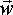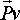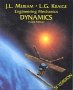# Physics - Kinematics - Combined Linear and Angular Acceleration

On this page we derive the rotation values from a point mass (particle). The point mass is not necessarily rotating about its own axis (although it could, subatomic paricles have spin). What we are interested in here is the contribution of the particle to the rotatinal properties of a bigger mass about some fixed point. If I am not making myself very clear try reading numerical methods.

The following pages will then go on to derive quantities for finite solid bodies by integrating across the volume. Most of these quantities are vectors of dimension 3 which has a component in the x,y and z directions. To denote a vector quantity we show an arrow above the quantity, for more information about vectors see here.

## Angular Acceleration of particle (α)

The linear acceleration of a particle on a solid body due to circular motion is:= a0 + α ×+ w × (w ×)

 where: other definitions symbol description type unitslinear acceleration of particle on a solid body vector m/s2 a0 linear acceleration of centre of mass of solid body vector m/s2 α angular acceleration of solid body bivector s-2 × cross multiplicationposition of particle relative to centre of mass vector m w angular velocity of solid body bivector s-1

As you can see from the equation it is made up of three parts, a linear acceleration due to the acceleration of the centre of mass and there are two parts due to the rotation as described here:

1. A linear acceleration due to centrifugal force|.
2. A linear acceleration due to any change in angular velocity
3. A linear acceleration due to the acceleration of the centre of mass.

Let us take a specific case, imagine that the particle is traveling in a circle around point. So in this case the linear acceleration will be made up of two components:

1. A linear acceleration due to centrifugal force, which is of magnitude w x (w x). The direction is toward the centre of the orbit, since w and r are at 90 degrees to each other this gives -* |w2|.
2. A linear acceleration due to any change in angular velocity d/dt xIn the above example I have shown the centre of the circle of rotation, and the point that we are measuring the angular acceleration about1, as being separate points.

But if we take the special case where we are measuring the angular acceleration about the centre of the circle of rotation, then the centrifugal acceleration is in the same direction asso the cross product is zero. So, in this case only, we only need to consider= d/DT

### Part due to angular acceleration

linear acceleration is the cross product of its angular acceleration, about a point, the with its distance from the point.= α x1

 where: other definitions symbol description type units α the instantaneous angular acceleration about Pc1 bivector radians s-2 x x is the cross product operator (see here for definition)1 the instantaneous position of the particle relative to point.-1 vector mthe instantaneous linear acceleration about Pc1 vector m/s2

Let us take a specific case, imagine that the particle is traveling in a circle around point. So in this case the linear acceleration will be made up of two components:

1. A linear acceleration due to centrifugal force, which is of magnitude rw^2 and with a direction toward the centre of the orbit.
2. A linear acceleration due to any change in angular velocityx d/DT

In the above example I have shown the centre of the circle of rotation, and the point that we are measuring the angular acceleration about1, as being separate points.

But if we take the special case where we are measuring the angular acceleration about the centre of the circle of rotation, then the centrifugal acceleration is in the same direction asso the cross product is zero. So, in this case only, we only need to consider α= d/dT

## Representing Angular Acceleration in program

Angular acceleration in 3D space can be held in a quaternion (see class sfrotation) or a matrix (see class sftransform). For an example of how this might be used in a scenegraph node, see here.

Book Shop - Further reading.

Where I can, I have put links to Amazon for books that are relevant to the subject, click on the appropriate country flag to get more details of the book or to buy it from them.Engineering Mechanics Vol 2: Dynamics - Gives theory for rigid dynamics, aims to allow prediction of effects of force and motion. Includes rotating frame of reference. Lots of colour diagrams, I guess its college / University level.

Commercial Software Shop

Where I can, I have put links to Amazon for commercial software, not directly related to the software project, but related to the subject being discussed, click on the appropriate country flag to get more details of the software or to buy it from them.Dark Basic Professional Edition - It is better to get this professional editionThis is a version of basic designed for building games, for example to rotate a cube you might do the following: make object cube 1,100 for x=1 to 360 rotate object 1,x,x,0 next x

This site may have errors. Don't use for critical systems.